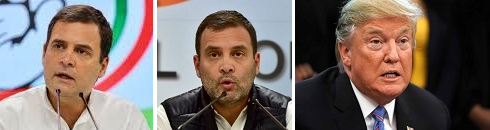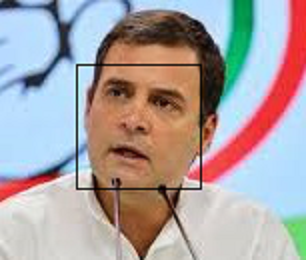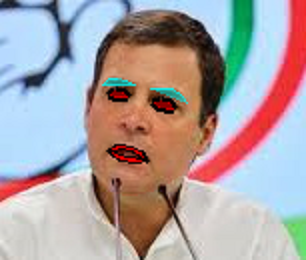# Introduction to Face Recognition

Featured on Hashnode

Subscribe to my newsletter and never miss my upcoming articles

Face recognition is one of the many wonders that AI research has brought forward to the world. It is a subject of curiosity for many techies — who would like to have a basic understanding of how things work. Let us take a dip into the subject, to see how things work.

## Intuition

To understand how a machine can recognize faces, we can start with asking ourselves — how do we recognize a face? Most images of human faces have two eyes, a nose, lips, forehead, chin, ears, hair… That rarely changes. Yet, faces are different from each other. What makes them different? At the same time, face of the same person changes with emotion, expression, age… In fact just change in orientation creates a different image. How do we identify a person in spite of all that?

On a gross level, one can say that there are some components of a face are related to age, emotion and orientation. While there are some other components that are stick to the person irrespective of the age, emotion, etc. Further, we can say that these components are not orthogonal or independent. We have all seen people who look alike sideways, but very different otherwise. Or, a kid in the family reminds people of his parents at that age, etc.

So, it is not so easy to logically identify these individual components. But, one can say that there are several overlapping components of the face — which are individually responsible for the perception of emotion, age and the person himself. Essentially, we know that there is “some relation that is too complex for logic” — that is where machine learning shows up!

## Training the Model

To train our neural network, we need a huge amount of data. Now, what kind of data do we use when we train a face recognition model? The model we generate should be able to measure similarity between two faces. It should be able to map an image of a face to a vector space such that images of the same person are closer to each other — irrespective of the other parameters. So our data set has to include several images of each person.

Like most other AI problems, these concepts are pretty old. But, the progress on face recognition was held back because of lack of data and processing power. With the boom in social media, we have obtained a huge amount of data — with a decent amount of labeling. Many researchers proposed different ways of going about this problem. Some started with the abstract — using unsupervised learning to start with just segregating and identifying different types of faces. Some took the down to earth approach with hard training the model based on each individual feature of the face. After working on various different approaches on a variety of data sets, we now have several solutions that offers accuracy better than human limits.

## face_recognition

When working on problems like this, it is best not to reinvent the wheel — we can’t! It is best to follow up with models that researchers have provided us. A lot of it is available in the open source as well. One such Python library is face_recognition. It works in a few steps:

• Identify a face in a given image
• Identify specific features in the face
• Generate a face encoding vector of 128 values

Based on this encoding, we can measure the similarity between two face images — that can tell us if they belong to the same person.

Let us check out an example.

## An Example

To check out an example, let us work on faces of two individuals. For making things easier, let us work with faces of two individuals known to most of us. Political leaders are popular all over the world, hence they using their photos.Disclaimer: These photos are used only for demonstration of the concepts. It does not in any way mean a positive or negative towards the individuals.

Let us start off with our notebooks. Install the face_recognition module.

``````!pip install face_recognition
``````

## Import Modules

Next, we import the required modules

``````import PIL.Image
import PIL.ImageDraw
import requests
from io import BytesIO

from IPython.display import display

import face_recognition
``````

## Load Image

Next, we load a picture. I have stored the images in my Github account. So we can read the raw images from the URL.

``````response = requests.get("https://raw.githubusercontent.com/solegaonkar/solegaonkar.github.io/master/img/rahul1.jpeg")
fr_image = face_recognition.load_image_file(BytesIO(response.content))
``````

# Identify Faces

Once we have loaded the face, let us have a look at parts of the face_recognition module. How does it identify faces?

``````face_locations = face_recognition.face_locations(fr_image)

number_of_faces = len(face_locations)
print("I found {} face(s) in this photograph.".format(number_of_faces))
``````

That gives us an output:

``````I found 1 face(s) **in** this photograph.</span>
``````

This means, the algorithm found just one face in the image. Let us have a look at the image and the face identified.

``````pil_image = PIL.Image.fromarray(fr_image)

for face_location in face_locations:
# Print the location of each face in this image. Each face is a list of co-ordinates in (top, right, bottom, left) order.
top, right, bottom, left = face_location
print("A face is located at pixel location Top: {}, Left: {}, Bottom: {}, Right: {}".format(top, left, bottom, right))
# Let's draw a box around the face
draw = PIL.ImageDraw.Draw(pil_image)
draw.rectangle([left, top, right, bottom], outline="black")
``````

This gives us an output:

``````A face **is** located at pixel location Top: 68, Left: 117, Bottom: 291, Right: 340</span>
``````

The above code also modifies the image to draw a rectangle around the face. Let us check if that worked well.That is good enough!

## Face Encodings

It is a face for us. But, for our algorithm, it is only an array of RGB values — that matches a pattern that the it has learnt from the data samples we provided to it.

For face recognition, the algorithm notes certain important measurements on the face — like the color and size and slant of eyes, the gap between eyebrows, etc. All these put together define the face encoding — the information obtained out of the image — that is used to identify the particular face.

To get a feel of what is read from the face, let us have a look at the encodings that we read.

``````face_encodings = face_recognition.face_encodings(fr_image)
face_encodings
``````

This prints out a huge array:

``````array([-0.10213576,  0.05088161, -0.03425048, -0.09622347, -0.12966095,
0.04867411, -0.00511892, -0.03418527,  0.2254715 , -0.07892745,
0.21497472, -0.0245543 , -0.2127848 , -0.08542262, -0.00298059,
0.13224372, -0.21870363, -0.09271716, -0.03727289, -0.1250658 ,
0.09436664,  0.03037129, -0.02634972,  0.02594662, -0.1627259 ,
-0.29416466, -0.12254384, -0.15237436,  0.14907973, -0.09940194,
0.02000656,  0.04662619, -0.1266906 , -0.11484023,  0.04613583,
0.1228286 , -0.03202137, -0.0715076 ,  0.18478717, -0.01387333,
-0.11409076,  0.07516225,  0.08549548,  0.31538364,  0.1297821 ,
0.04055009,  0.0346106 , -0.04874525,  0.17533901, -0.22634712,
0.14879328,  0.09331974,  0.17943285,  0.02707857,  0.22914577,
-0.20668915,  0.03964197,  0.17524502, -0.20210043,  0.07155308,
0.04467429,  0.02973968,  0.00257265, -0.00049853,  0.18866715,
0.08767469, -0.06483966, -0.13107982,  0.21610288, -0.04506358,
-0.02243116,  0.05963502, -0.14988004, -0.11296406, -0.30011353,
0.07316103,  0.38660526,  0.07268623, -0.14636359,  0.08436179,
0.01005938, -0.00661338,  0.09306039,  0.03271955, -0.11528577,
-0.0524189 , -0.11697718,  0.07356471,  0.10350288, -0.03610475,
0.00390615,  0.17884226,  0.04291092, -0.02914601,  0.06112404,
0.05315027, -0.14561613, -0.01887275, -0.13125736, -0.0362937 ,
0.16490118, -0.09027836, -0.00981111,  0.1363602 , -0.23134531,
0.0788044 , -0.00604869, -0.05569676, -0.07010217, -0.0408107 ,
-0.10358225,  0.08519378,  0.16833456, -0.30366772,  0.17561394,
0.14421709, -0.05016343,  0.13464174,  0.0646335 , -0.0262765 ,
0.02722404, -0.06028951, -0.19448066, -0.07304715,  0.0204969 ,
-0.03045784, -0.02818791,  0.06679841])
``````

Each of these numbers represents an orthogonal component of the face encoding.

## Similarity

Let us now look into the next step — identifying similarity between faces. For that, we need to load more images.

Let us start by loading the three images. As we load the images, we also find the faces and then the face encoding.

``````
response = requests.get("https://raw.githubusercontent.com/solegaonkar/solegaonkar.github.io/master/img/rahul1.jpeg")
image_of_person_1 = face_recognition.load_image_file(BytesIO(response.content))
face_locations = face_recognition.face_locations(image_of_person_1)
person_1_face_encoding = face_recognition.face_encodings(image_of_person_1, known_face_locations=face_locations)

response = requests.get("https://raw.githubusercontent.com/solegaonkar/solegaonkar.github.io/master/img/rahul2.jpg")
image_of_person_2 = face_recognition.load_image_file(BytesIO(response.content))
face_locations = face_recognition.face_locations(image_of_person_2)
person_2_face_encoding = face_recognition.face_encodings(image_of_person_2, known_face_locations=face_locations)

response = requests.get("https://raw.githubusercontent.com/solegaonkar/solegaonkar.github.io/master/img/trump.jpg")
image_of_person_3 = face_recognition.load_image_file(BytesIO(response.content))
face_locations = face_recognition.face_locations(image_of_person_3)
person_3_face_encoding = face_recognition.face_encodings(image_of_person_3, known_face_locations=face_locations)
``````

Now, identifying the similarity is not difficult. The face_recognition module offers a simple API for doing that.

``````face_recognition.compare_faces([person_1_face_encoding,person_3_face_encoding], person_2_face_encoding, tolerance=0.08)
``````

This method checks each of the component of the two faces being compared, and tells us if the component at hand varies within the tolerance limits. The above command presents an output like this:

``````[array([ True,  True,  True,  True,  True,  True,  True,  True,  True,
True,  True,  True,  True,  True,  True,  True,  True,  True,
True,  True,  True,  True,  True,  True,  True,  True,  True,
True,  True,  True,  True,  True,  True,  True,  True,  True,
True,  True,  True,  True, False,  True,  True,  True,  True,
True,  True,  True,  True,  True,  True,  True,  True, False,
True,  True,  True,  True,  True,  True,  True,  True,  True,
True,  True,  True,  True,  True, False,  True,  True,  True,
True,  True,  True,  True,  True,  True,  True,  True, False,
True,  True,  True,  True,  True,  True,  True,  True,  True,
True,  True,  True, False,  True,  True,  True,  True,  True,
True,  True,  True,  True,  True,  True,  True,  True,  True,
True,  True,  True,  True,  True,  True,  True,  True,  True,
True,  True,  True,  True,  True,  True,  True,  True, False,
True,  True]),
array([ True,  True,  True,  True,  True,  True, False, False, False,
True,  True,  True, False,  True,  True,  True, False,  True,
False,  True,  True,  True,  True, False,  True,  True,  True,
False,  True,  True,  True, False,  True,  True,  True,  True,
True,  True,  True,  True, False,  True, False,  True,  True,
True,  True,  True, False,  True, False,  True,  True,  True,
False, False,  True,  True,  True,  True,  True, False,  True,
False, False, False, False,  True, False,  True, False,  True,
False,  True,  True,  True,  True, False,  True,  True,  True,
True,  True,  True, False,  True,  True,  True, False,  True,
True, False,  True,  True,  True,  True,  True,  True,  True,
True,  True,  True,  True,  True, False, False,  True,  True,
False, False, False,  True,  True, False,  True,  True,  True,
True,  True,  True,  True,  True,  True, False, False,  True,
True,  True])]
``````

These two arrays denote the similarity of the given image (in second parameter) with each of known face encodings in the list provided (in first parameter).

We can see that the first array shows a lot more similarity. That correctly identifies the person.

## Digital Makeup

If you like fun, we can use the face recognition library to do a lot more. We have an API that can help us identify individual features on the face.

``````face_landmarks_list = face_recognition.face_landmarks(fr_image)
print(face_landmarks_list)
``````

This gives us the long list of the curves for each of the individual features.

``````[{
'chin': [(46, 47), (45, 54), (44, 62), (44, 69), (44, 77), (46, 84), (49, 91), (54, 95), (61, 97), (68, 97), (76, 95), (84, 91), (90, 87), (94, 81), (97, 75), (99, 68), (101, 60)],
'left_eyebrow': [(51, 42), (54, 39), (58, 39), (63, 40), (67, 42)],
'right_eyebrow': [(75, 44), (80, 44), (86, 44), (90, 47), (93, 51)],
'nose_bridge': [(70, 48), (68, 52), (67, 56), (66, 60)],
'nose_tip': [(60, 64), (62, 65), (65, 67), (68, 66), (71, 66)],
'left_eye': [(55, 47), (57, 45), (61, 46), (63, 48), (60, 48), (57, 48)],
'right_eye': [(77, 51), (80, 50), (84, 51), (86, 54), (83, 54), (79, 53)],
'top_lip': [(54, 75), (58, 72), (61, 72), (64, 73), (66, 73), (70, 75), (73, 80), (71, 79), (66, 75), (63, 75), (61, 74), (56, 75)],
'bottom_lip': [(73, 80), (68, 81), (64, 81), (62, 80), (60, 80), (57, 78), (54, 75), (56, 75), (60, 77), (63, 78), (65, 78), (71, 79)]
}]
``````

We can apply a digital makeup to this image.

``````for face_landmarks in face_landmarks_list:
pil_image = PIL.Image.fromarray(fr_image)
d = PIL.ImageDraw.Draw(pil_image, 'RGBA')

# Make the eyebrows into a nightmare
d.line(face_landmarks['left_eyebrow'], fill=(0, 0, 0, 255), width=3)
d.line(face_landmarks['right_eyebrow'], fill=(0, 0, 0, 255), width=3)
d.polygon(face_landmarks['left_eyebrow'], fill=(0, 0, 0, 255))
d.polygon(face_landmarks['right_eyebrow'], fill=(0, 0, 0, 255))

# Gloss the lips
d.line(face_landmarks['top_lip'], fill=(0, 0, 0, 255), width=10)
d.line(face_landmarks['bottom_lip'], fill=(0, 0, 0, 255), width=10)

d.polygon(face_landmarks['bottom_lip'], fill=(255, 0, 0, 255))
d.polygon(face_landmarks['top_lip'], fill=(255, 0, 0, 255))
d.line(face_landmarks['top_lip'], fill=(0, 0, 0, 255), width=2)
d.line(face_landmarks['bottom_lip'], fill=(0, 0, 0, 255), width=2)

# Chin
d.polygon(face_landmarks['chin'], fill=(255, 0, 0, 16))

# Apply some eyeliner
d.line(face_landmarks['left_eye'] + [face_landmarks['left_eye']], fill=(10, 0, 0, 255), width=6)
d.line(face_landmarks['right_eye'] + [face_landmarks['right_eye']], fill=(10, 0, 0, 255), width=6)

# Sparkle the eyes
d.polygon(face_landmarks['left_eye'], fill=(255, 0, 0, 200))
d.polygon(face_landmarks['right_eye'], fill=(255, 0, 0, 200))

display(pil_image)
``````

And this is what we get.### Comments (2)

Hello Vikas, The blog gives information about face detection and not face recognition so kindly change the title name.

Aman, thanks for your comment. But I would suggest that you read the full blog before concluding. It does talk about recognizing faces based on similarly - using the face_recognition librery